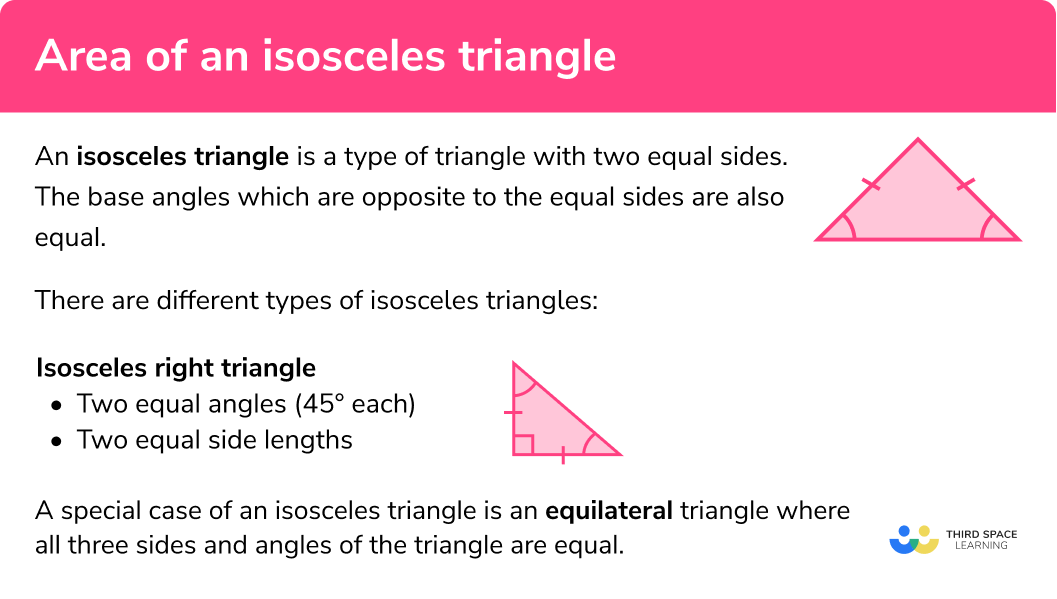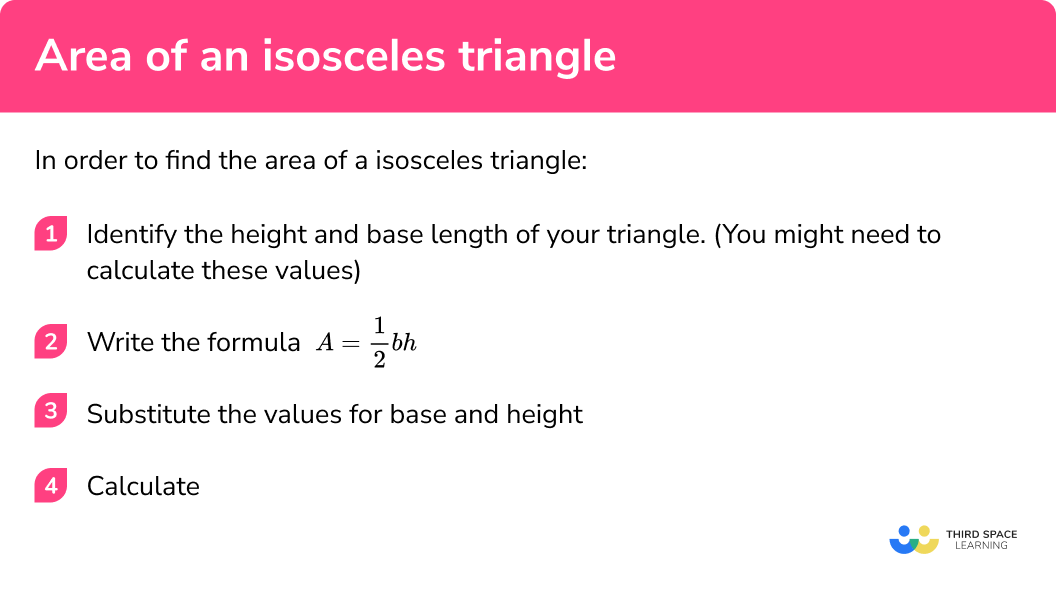GCSE Maths Geometry and Measure Area

Area Of An Isosceles Triangle

# Area Of An Isosceles Triangle

Here we will learn about the area of an isosceles triangle including how to find the area of an isosceles with given lengths and how to calculate those lengths if they are not given.

There are also area of a triangle worksheets based on Edexcel, AQA and OCR exam questions, along with further guidance on where to go next if you’re still stuck.

## What is an isosceles triangle?

An isosceles triangle is a type of triangle with two equal sides. The base angles which are opposite to the equal sides are also equal.

There are different types of isosceles triangles:

Isosceles right triangle

-two equal angles (45° each)

-two equal side lengths

A special case of an isosceles triangle is an equilateral triangle where all three sides and angles of the triangle are equal.

### What is an isosceles triangle?### How to name a triangle

We can identify a triangle by putting a capital letter on each vertex (corner).

We can then refer to each of the sides of the triangle by using two letters to describe where the line starts and ends.

We can refer to the entire triangle by using all three letters.

E.g.

Name of sides:
side AB, side AC, side BC

Name of triangle:
triangle ABC

### How do we find the area of a triangle?

In order to find the area of a triangle, we need to start with the area of a rectangle.

To find the area of a rectangle you must multiply adjacent sides together.

The area of the rectangle below would be calculated by multiplying the base x height
(b x h).

We can create an isosceles triangle by drawing two sides from the midpoint of a side of the rectangle to the corners.

The rectangle has been split into an isosceles triangle and two congruent (identical) right angled triangles. If we combine the area of the two right angled triangles they will form the pink isosceles triangle.

The area of the isosceles triangle is exactly half the area of the rectangle.

### Area of an isosceles triangle formula

$\text { Area of a triangle }=\frac{\text { base } \times \text { height }}{2}$

This can be shortened to

$A=\frac{1}{2} b h$

where b is the base length and h is the height of the triangle.

To find the area of an isosceles triangle when only the side lengths have been given, we will need to calculate the height of the triangle using Pythagoras’ Theorem

Finding the height using Pythagoras’ Theorem

Let the base of the isosceles triangle be b and the two equal sides be l.

Drawing a perpendicular line from the top vertex to the base forms the height, h, and also forms a right angled triangle with hypotenuse l and short sides h and \cfrac{1}{2} \, b.

h=\sqrt{{{l}^{2}}-{{\left( \cfrac{1}{2} \, b \right)}^{2}}}

We can also find the area of the isosceles triangle using the area of a triangle formula

Area=\cfrac{1}{2} \, ab\sin C

If we know (or can find) the two equal sides, l and the angle between them, \theta , the formula would give the area as

A=\cfrac{1}{2} \, {{l}^{2}}\sin \left( \theta \right)

## How to find the area of an isosceles triangle

In order to find the area of a isosceles triangle:

1Identify the height and base length of your triangle if given. (You might need to calculate these values)

2Write the appropriate formula
A=\cfrac{1}{2} \,b h or A=\frac{1}{2}{{l}^{2}}\sin \left( \theta \right)

3Substitute the values into the formula

4Calculate

### How to find the area of an isosceles triangle## Related lessons on area

Area of isosceles triangles is part of our series of lessons to support revision on area. You may find it helpful to start with the main area lesson for a summary of what to expect, or use the step by step guides below for further detail on individual topics. Other lessons in this series include:

## Area of isosceles triangles examples

### Example 1: given base length and height

Find the area of the triangle below:

1. Identify the height and base length of the triangle if given.

h = 6cm

b = 10cm

2Write down the appropriate formula

$A=\frac{1}{2} b h$

3Substitute the values into the formula

\begin{aligned} A &=\frac{1}{2} b h \\ &=\frac{1}{2}(6)(10) \end{aligned}

4Calculate

\begin{aligned} A &=\frac{1}{2} b h \\ &=\frac{1}{2}(6)(10)\\ &=30cm^2 \end{aligned}

### Example 2: given base length and height

Calculate the area of the triangle below:

Identify the height and base length of the triangle if given.

Write down the appropriate formula

Substitute the values into the formula.

Calculate

### Example 3: worded question

Shown below is a triangular shaped field. Each cow needs 2000m^{2} to graze. How many cows can fit into this field?

Identify the height and base length of the triangle if given.

Write down the appropriate formula

Substitute the values into the formula.

Calculate

### Example 4: missing height

Find the area of the triangle below:

Identify the height and base length of the triangle if given.

Write down the appropriate formula

Substitute the values into the formula.

Calculate

### Example 5: compound shapes

Below is the floor plan for a new house. Calculate the area of the plan.

Identify the height and base length of the triangle if given.

Write down the appropriate formula

Substitute the values into the formula.

Calculate

### Example 6: Using the ½ab sin C formula

Below shows an isosceles triangle with two sides of length 10 \, cm and a base angle of 75^{\circ}.

Find the area.

Identify the height and base length of your triangle if given. (You might need to calculate these values)

Write down the appropriate formula

Substitute the values into the formula.

Calculate

### How to find a missing side length given the area

Sometimes a question might give you the area and ask you to work out the height or missing length. In order to do this you must rearrange the formula.

To find a missing length given the area:

1Rearrange the formula

2Substitute in the values you know

3Calculate

Step by step guide: Rearranging equations

### Example 7: calculating base length

Triangle ABC is an isosceles triangle with an area of 18cm^{2}. The height of the triangle is 6cm. Find the length of the base of the triangle.

Rearrange the formula

Now substitute the given values.

Calculate

### Common misconceptions

• Identifying the correct information to use

A question may give extra information that is not needed to answer it. Carefully identify the relevant pieces of information.

E.g.
To calculate the area here we only need the base and height.

Base= 12cm

Height = 8cm

$Area = \frac{12 \times 8}{2} = 48cm^{2}$

We can ignore the values of the other sides (10cm)

• Units

It is a common error to forget the units for area in the final answer. When calculating area, your answer must always have units squared.

### Practice area of an isosceles triangle questions

1. Find the area of the triangle below90cm^{2}45cm^{2}180cm^{2}21cm^{2}Using the lengths for base and height, the calculation we must perform is \frac{1}{2} \times 15 \times 6

2. Find the area of the triangle below giving your answer in cm^{2}225cm^{2}2250cm^{2}22500cm^{2}45000cm^{2}After converting the height to the appropriate units, the calculation becomes \frac{1}{2} \times 300 \times 150

3. Shown below is a triangular shaped chicken enclosure cage. Each chicken needs 8m^{2} to roam around. How many chickens can fit into this enclosure?125 chickens15 chickens16 chickens30 chickensThe area of the enclosure is \frac{1}{2} \times 20 \times 12.5 = 125m^{2}. We then consider multiples of 8 to work out how many chickens will fit.

Or use a division method: 125 \div 8 = 15.625

So 15 chickens will fit.

4. Find the area of the triangle below (give your answer to two decimal places)18.00cm^{2}9.00cm^{2}8.71cm^{2}17.42cm^{2}The height of the triangle can be found using Pythagoras’ Theorem.

Height = \sqrt{6^{2}-1.5^{2}} Height = 5.809…

The area of the triangle is then given by \frac{1}{2} \times 3 \times 5.809…24.6m^{2}18.3m^{2}12.3m^{2}19.4m^{2}The shape can be split into a rectangle and a triangle.

The area of the rectangle is 3 \times 4 = 12m^{2}

The area of the triangle is \frac{1}{2} \times 3 \times 4.2 = 6.3m^{2}

The total area is 12 + 6.3 = 18.3m^{2}

6. Triangle MNP is an isosceles triangle with an area of 20cm^{2} . The base length of the triangle is 4cm . Find the height of the triangle.

80cm5cm2.5cm10cmStarting with the formula and the information we know already,

Area = \frac{1}{2}  \times base \times height

20 = \frac{1}{2} \times 4  \times height

20 = 2  \times height

So the height is 10cm

### Area of an isosceles triangle GCSE questions

1. This pattern is made from three identical isosceles triangles. Find the total area.(3 marks)

10 \div 2 = 5

(1)

One triangle:

\begin{aligned} A&= \frac{1}{2} \times 5 \times 12\\ A&=30cm^{2} \end{aligned}

(1)

Total area:

3 \times 30 = 90cm^{2}

(1)

2. (a) Find the area of the following isosceles triangle:(b) The isosceles triangle below has the same area as the triangle in
part (a).Work out the height of this triangle.

(3 marks)

\begin{aligned} A&= \frac{1}{2} \times 8 \times 12\\ A&=48cm^{2} \end{aligned}

(1)

Rearrange area of triangle:

\begin{aligned} A &= \frac{1}{2} bh \\ 2A &= bh\\ h & = \frac{2A}{b} \end{aligned}

Substitute in values:

h= \frac{2 \times 48}{16}

(1)

\begin{array}{l} h= \frac{96}{16}\\ h=6cm \end{array}

(1)

3. Calculate the area of the isosceles triangle.(4 marks)

Find height of triangle using Pythagoras:

h^{2}=5^{2}-3^{2}

(1)

\begin{aligned} h^{2}&=16\\ h&=4 \end{aligned}

(1)

A=\frac{1}{2} \times 6 \times 4

(1)

A=12cm^{2}

(1)

## Learning checklist

You have now learned how to:

•  Apply formula to calculate and solve problems involving the area of triangles
•  Use Pythagoras’ Theorem to solve problems involving triangles

## Still stuck?

Prepare your KS4 students for maths GCSEs success with Third Space Learning. Weekly online one to one GCSE maths revision lessons delivered by expert maths tutors.

Find out more about our GCSE maths tuition programme.